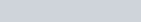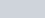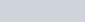Construct an isosceles triangle whose base is 8 cm and altitude
Question:

Construct an isosceles triangle whose base is 8 cm and altitude 4 cm and then another triangle whose sides are 3/2 times the corresponding sides of the isosceles triangle.

Solution:

Given that

Construct an isosceles triangle $\mathrm{ABC}$ in which $A B=B C=6 \mathrm{~cm}$ and altitude $=4 \mathrm{~cm}$ then another triangle similar to it whose sides are $\frac{3}{2}$ of the corresponding sides of $\triangle A B C$.

We follow the following steps to construct the givenStep of construction

Step: I- First of all we draw a line segment AB = 6 cm.

Step: II- With as centre and radius = BC = 6 cm, draw an arc.

Step: III- From point A and B constructwhich cut the line BS at point C

Step: IV -Join AC to obtain.

Step: V- Below AB, makes an acute angle.

Step: VI -Along $A X$, mark off five points $A_{1}, A_{2}$ and $\mathrm{A}_{3}$ such that $A A_{1}=A_{1} A_{2}=A_{2} A_{3}$

Step: VII- Join $A_{2} B$.

Step: VIII -Since we have to construct a triangle $\triangle A Q R$ each of whose sides is $(1.5$ times $=3 / 2)$ of the corresponding sides of $\triangle A B C$.

So, we draw a line $A_{3} Q$ on $A X$ from point $A_{3}$ which is $A_{3} Q \| A_{2} B$, and meeting $A B$ at $Q$.

Step: IX -From $Q$ point draw $Q R \mid B C$, and meeting $A C$ at $R$

Thus, $\triangle A Q R$ is the required triangle, each of whose sides is $(3 / 2)$ of the corresponding sides of $\triangle A B C$.